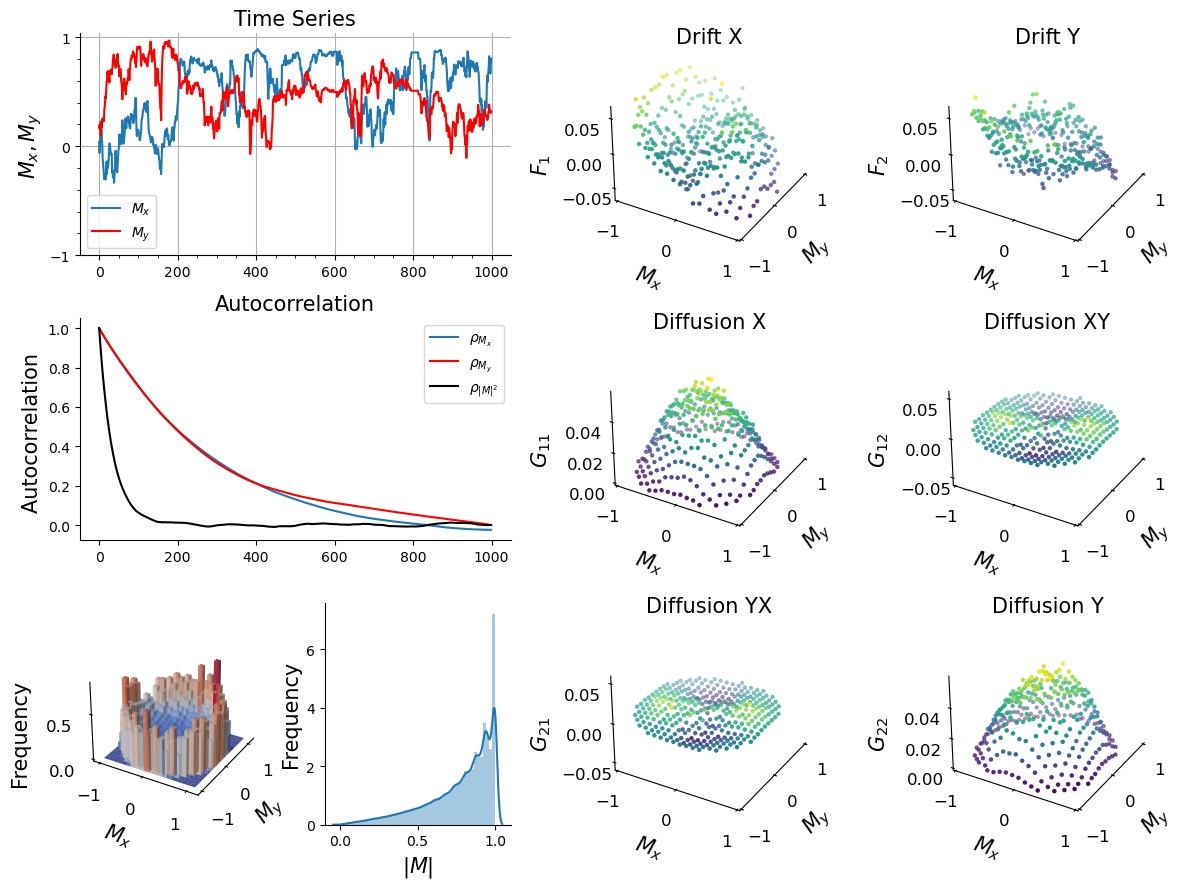# PyDaddy (Python Data-Driven Dynamics)

PyDaddy is a comprehensive and easy to use python package to discover data-derived stochastic differential equations from time series data. PyDaddy takes the time series of state variable $$x$$, scalar or 2-dimensional vector, as input and discovers an SDE of the form:

$\frac{dx}{dt} = f(x) + g(x) \cdot \eta(t)$

where $$\eta(t)$$ is uncorrelated white noise. The function $$f$$ is called the drift, and governs the deterministic part of the dynamics. $$g^2$$ is called the diffusion and governs the stochastic part of the dynamics.An example summary plot generated by PyDaddy, for a vector time series dataset.

PyDaddy also provides a range of functionality such as equation-learning for the drift and diffusion functions using sparse regresssion and a suite of diagnostic functions.

## Get Started

• To take PyDaddy for a walk, see the tutorial notebooks. The notebooks can be executed online on Google Colab; no installation is necessary!

• To install PyDaddy on your system, see installation instructions.

• See the usage guide and advanced usage tips for detailed instructions and tips on how to use PyDaddy.

## Citation

If you are using this package in your research, please cite the repository and the associated paper as follows:

Nabeel, A., Karichannavar, A., Palathingal, S., Jhawar, J., Danny Raj, M., & Guttal, V. (2022). PyDaddy: A Python Package for Discovering SDEs from Time Series Data (Version 0.1.5) [Computer software]. https://github.com/tee-lab/PyDaddy

Nabeel, A., Karichannavar, A., Palathingal, S., Jhawar, J., Danny Raj, M., & Guttal, V. (2022). PyDaddy: A Python package for discovering stochastic dynamical equations from timeseries data. arXiv preprint arXiv:2205.02645.